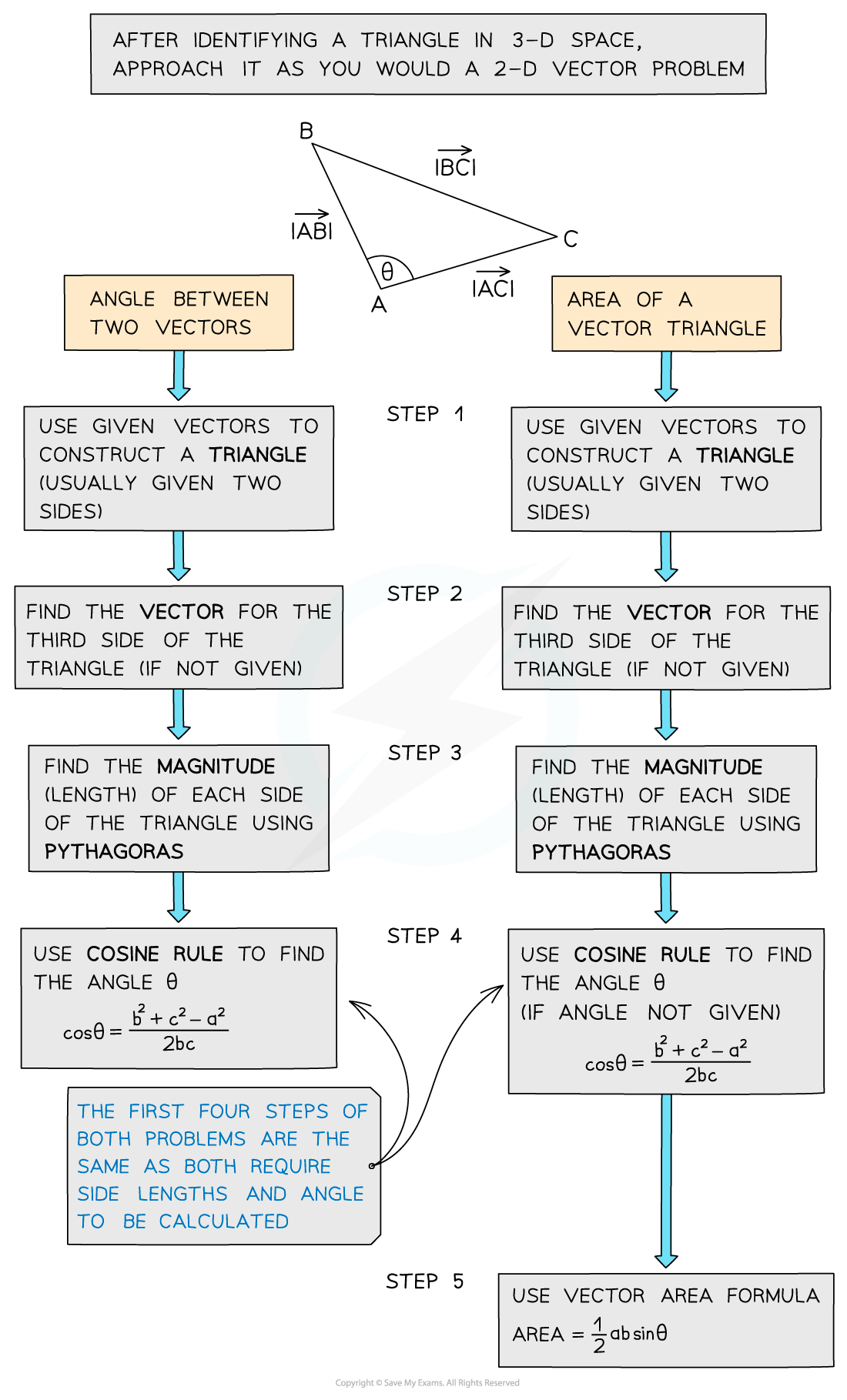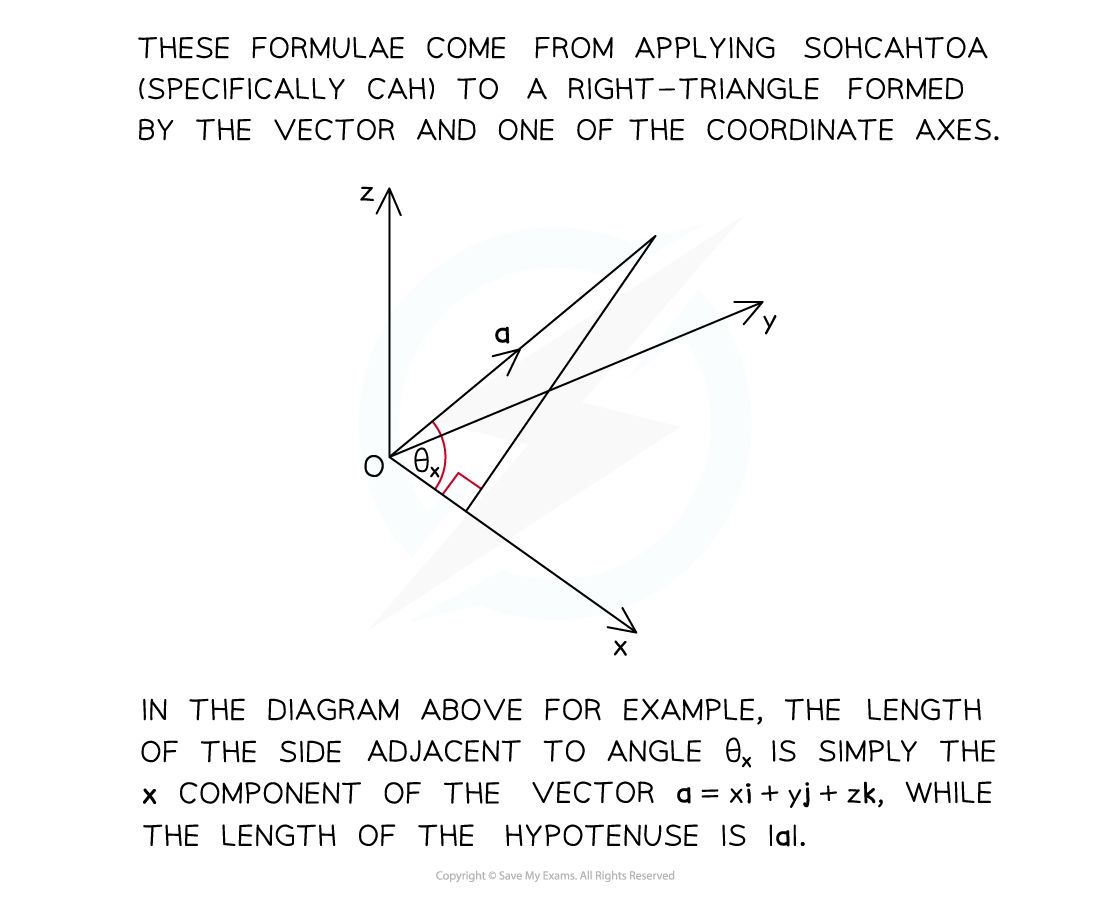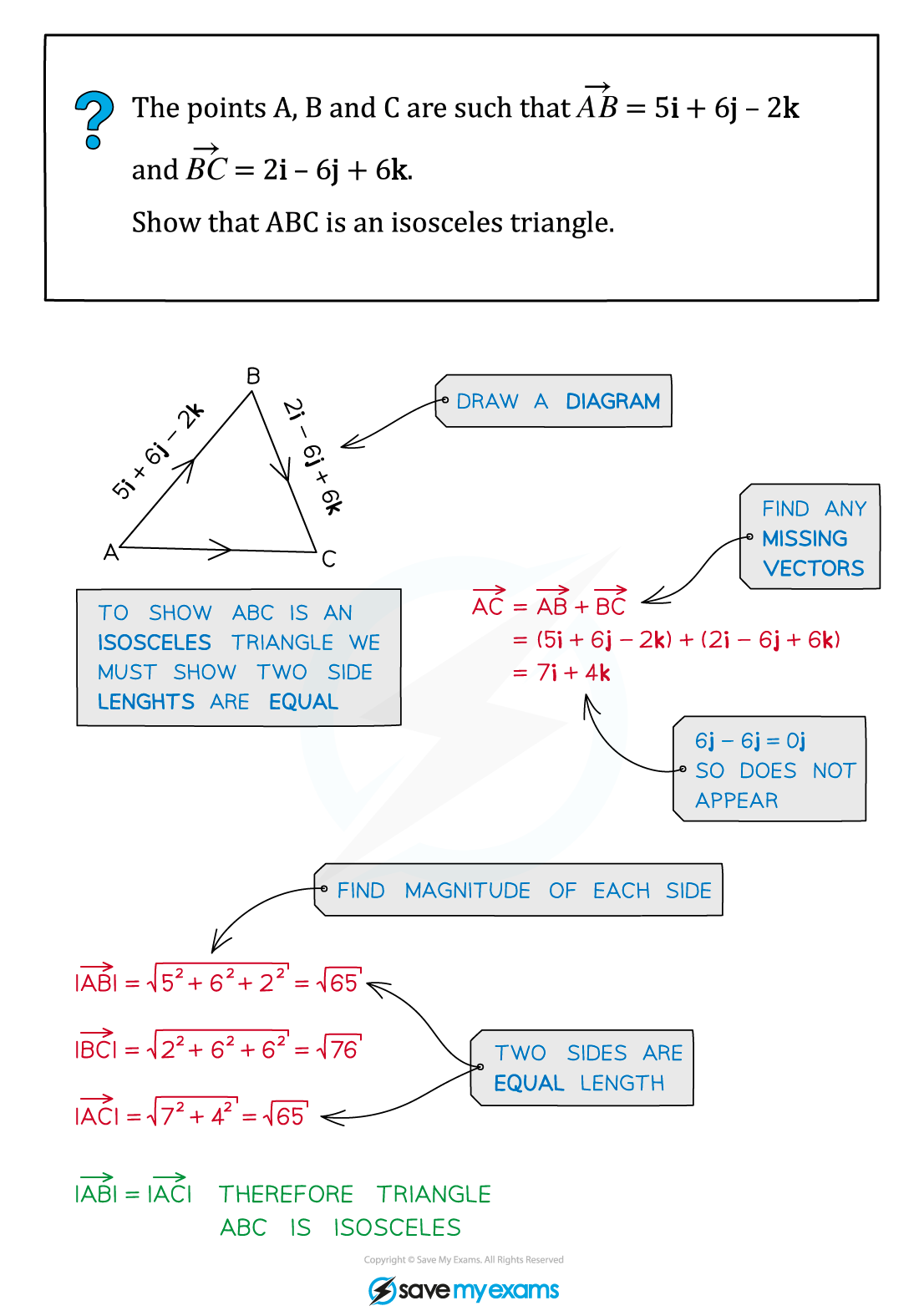# AQA A Level Maths: Pure复习笔记11.2.2 Problem Solving using 3D Vectors

### Problem Solving using 3D Vectors

#### Problem-solving with 3-D vectors

• 3-D vector problems can be solved using the same principles as 2-D vector problems (see Problem Solving using Vectors)
• Vectors can be used to prove two lines are parallel, to show points are collinear (lie on the same straight line) or to find missing vertices of a given shape#### Using trig in 3-D vector problems

• 3-D vector problems can also involve using trigonometry to:
• find the angle between two vectors using Cosine Rule
• find the area of a triangle using a variation of Area Formula• You can find the angle between a vector and any one of the coordinate axes by using the following formulae• These formulae are not in the exam formulae book
• However, they may be derived using SOHCAHTOA as shown in the diagram
• The formulae also work for vectors in two dimensions

#### Exam Tip

• If there is a diagram, labelling all known vectors and quantities will help, and don’t worry about trying to make your diagram 3-D, as long as you label it well.

#### Worked Example